Relative Frequency Theory of probability, Business Mathematics and Statistics

# Relative Frequency Theory of probability, Business Mathematics and Statistics - Business Mathematics and Statistics - B Com

Relative Frequency Theory of probability : Classical approach is useful for solving problems involving game of chances—throwing dice, coins, etc. but if applied to other types of problems it does not provide answers. For instance, if a man jumps from a height of 300 feet, the probability of his survival will, not be 50%, since survival and death are not equally alike.
Similarly, the prices of shares of a Joint Stock Company have three alternatives i.e. the prices may remain constant or prices may go up or prices may go down. Thus, the classical approach fails to answe questions of these type.
If we toss a coin 20 times, the classical probability suggests that we; should have heads ten times. But in practice it may not be so. These empirical approach suggests, that if a coin is tossed a large number of time, say, 1,000 times, we can expect 50% heads and 50% tails. Vor Miscs explained, “If the experiment berepeated a large number of times under essentially identical conditions, the limiting value of the ratio of the number of times the event A happens to the total, number of trials.of the experiments as the number of trials increases indefinitely,  is called the probability of the occurrence of A”.
Thus,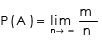The happening of an event is determined on the basis of past experience or on the basis of relative frequency of success in the past. For instance, a machine produces 10% unacceptable articles of the total output. On the basis of such experience or experiments, we may arrive at that (i) the relative frequency obtained on the basis of past experience can be shown to come very close to the classical probability. For example, as said earlier, a coin is tossed for 6 times, we may not get exactly 3 heads and 3 tails. But, the coin is tossed for larger number of times, say 10,000 times, we can expect heads and tails very close to 50% (ii) There are certain laws, according to which the ‘occurrence’ or ‘non-occurrence of the events take place. Posterior probabilities, also called Empirical Probabilities are based on experiences of the past and on experiments conducted. Thus, relative frequency can be termed as a measure of probability and it is calculated on the basis of empirical or statistical findings. For instance if a machine produces 100 articles in the past, 2 particles were found to be defective, then the probability of the defective articles is 2/100 or 2%.

Limitations of Relative Frequency Theory of Probability:

1. The experimental conditions may not remain essentially homogeneous and identical in a large number of repetitions of the experiment.

2. The relative frequency —, may not attain a unique value no matter however large N may be.

3. Probability P(A) defined can never be obtained in practice. We can only attempt at a close estimate of P(A) by making N sufficiently large.

Example 6:
An urn contains 8 white and 3 red balls. If two balls are drawn at random, find the probability that (a) both are white, (b) both are red and (c) one is of each colour.
Solution :
Total number of balls in the urn = 8 + 3 =11 Two balls can be drawn out of 11 balls in 11C2 ways.
Exhaustive number of cases =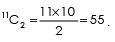(a) Two white balls to be drawn out of 8 white, can be done in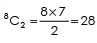ways.

The probability that both are white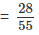(b) Two red balls to be drawn out of 3 red balls can be done in 3C2=3 ways.
Hence, the probability that both are red ​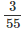(c) The number of favourable cases for drawing one white ball and one red ball is 8C1x3C1= 8 x 3 = 24.

Therefore, the probability (one red and one white)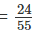Example 7 :
Tickets are numbered from 1 to 100. They are well shuffled and a ticket is drawn at random. What is the probability that the drawn ticket has :

(a) an even number,
(b) a number 5 or a multiple of 5,
(c) a number which is greater than 75,
(d) a number which is a square ?

Solution :
(a) The total number of exhaustive, mutually exclusive and equal cases is 100. There are 50 even numbered tickets.
Therefore, favourable cases to the event is 50.
Therefore, the probability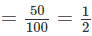(b) Suppose A denotes the number of happenings that the drawn ticket has a number 5 or a multiple of 5. These are 20 cases i. e., 5, 10, 15, 20,...100.
Therefore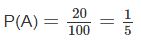(c) There are 25 cases, which have a number greater than 75. Say A will denote it.
Therefore,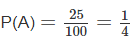(d) There are 10 favourable cases which give squares between 1 and 100 i.e., 1, 4, 9, 16, 25, 36, 49, 64, 81, 100.
Therefore,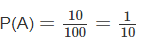Example 8 :
Four cards are drawn from a pack of 52 cards without replacement. What is the probability that they are all of different suits ?
Solution :
The required probability would be :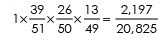The document Relative Frequency Theory of probability, Business Mathematics and Statistics | Business Mathematics and Statistics - B Com is a part of the B Com Course Business Mathematics and Statistics.
All you need of B Com at this link: B Com

115 videos|142 docs

## FAQs on Relative Frequency Theory of probability, Business Mathematics and Statistics - Business Mathematics and Statistics - B Com

 1. What is the Relative Frequency Theory of probability?Ans. The Relative Frequency Theory of probability is a statistical concept that suggests that the probability of an event occurring can be determined by observing the relative frequency of its occurrence in a large number of trials or experiments. It states that as the number of trials increases, the observed relative frequency of an event will approach its theoretical probability.
 2. How is the Relative Frequency Theory applied in Business Mathematics and Statistics?Ans. In Business Mathematics and Statistics, the Relative Frequency Theory is applied to make predictions and analyze data. It helps in determining the probability of certain events happening in a business context, such as the probability of a product being defective, the likelihood of meeting sales targets, or the probability of a customer returning for repeat purchases. By analyzing the relative frequency of these events in past data, businesses can make informed decisions and develop strategies.
 3. How is the concept of relative frequency calculated?Ans. The concept of relative frequency is calculated by dividing the frequency of a particular event by the total number of trials or observations. For example, if a coin is flipped 100 times and it lands on heads 60 times, the relative frequency of heads would be 60/100 = 0.6 or 60%.
 4. What are the advantages of using the Relative Frequency Theory in probability calculations?Ans. The Relative Frequency Theory offers several advantages in probability calculations. Firstly, it allows for the use of empirical data, making the calculations more accurate and reliable. Secondly, it provides a practical approach to determining probabilities based on observed frequencies, which is particularly useful when dealing with real-world events. Additionally, by analyzing relative frequencies over time, businesses can identify trends and patterns, enabling them to make more informed decisions.
 5. Are there any limitations or drawbacks to using the Relative Frequency Theory?Ans. While the Relative Frequency Theory is a valuable tool in probability calculations, it does have some limitations. One limitation is that it requires a large number of trials or observations to obtain accurate results. If the sample size is too small, the relative frequencies may not reflect the true probabilities. Another limitation is that it assumes that the underlying conditions and factors influencing the events remain constant, which may not always be the case in real-world scenarios. Therefore, it is important to consider these limitations when applying the Relative Frequency Theory in business mathematics and statistics.

115 videos|142 docsExplore Courses for B Com examSignup to see your scores go up within 7 days! Learn & Practice with 1000+ FREE Notes, Videos & Tests.
10M+ students study on EduRev
Track your progress, build streaks, highlight & save important lessons and more!
Related Searches

,

,

,

,

,

,

,

,

,

,

,

,

,

,

,

,

,

,

,

,

,

,

,

,

;